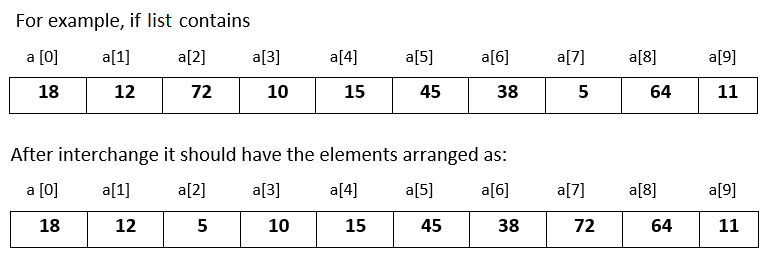# Input 10 numbers in integer list and swap the largest and smallest number in Python

###### Lists - Question 6

In this question, we will see how to input 10 numbers in integer list and interchange the largest number with the smallest number within the list and print the modified list in Python programming. To know more about lists click on the lists lesson.

Q6) Write a program in Python to input 10 numbers in integer list and interchange the largest number with the smallest number within the list and print the modified list. Assume that there is only one largest and smallest number.#### Program

``````ln=0; lnp=0; sn=0; snp=0
a=list()

print('Enter 10 numbers')
for i in range(10):
a.append(int(input()))

for i in range(10):
if i==0:
ln=a[i]
sn=a[i]
lnp=i
snp=i

elif a[i]>ln:
ln=a[i]
lnp=i

elif a[i]<sn:
sn=a[i]
snp=i

a[lnp]=sn
a[snp]=ln

print('\List after interchanging largest with the smallest');
for n in a:
print(n,end=' ')``````

#### Output

```Enter 10 numbers
18
12
72
10
15
45
38
5
64
11

Array after interchanging largest with the smallest
18 12 5 10 15 45 38 72 64 11```